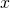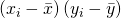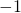Want to share your content on R-bloggers? click here if you have a blog, or here if you don't.

You may have misread the title as the old correlation does not imply causation mantra, but the opposite is also true! If you don’t believe me, read on…

First I want to provide you with some intuition on what correlation is really all about! For many people (and many of my students for sure) the implications of the following formula for the correlation coefficientof two variablesandare not immediately clear:In fact the most interesting part is this:. We see a product of two differences. The differences consist of the data points minus the respective means (average values): in effect this leads to the origin being moved to the means of both variables (as if you moved the crosshair right into the centre of all data points).

There are now four possible quadrants for every data point: top or bottom, left or right. Top right means that both differences are positive, so the result will be positive too. The same is true for the bottom left quadrant because minus times minus equals plus (it often boils down to simple school maths)! The other two quadrants give negative results because minus times plus and plus times minus equals minus.

After that we sum over all products and normalize them by dividing by the respective standard deviations (how much the data are spread out), so that we will only get values betweenand.

Let us see this in action with an example. First we define a helper function for visualizing this intuition:

cor.plot <- function(data) {
x_mean <- mean(data[ , 1])
y_mean <- mean(data[ , 2])
plot(data, type = "n") # plot invisibly
limits = par()$usr # limits of plot # plot correlation quadrants rect(x_mean, y_mean, limits, limits, col = "lightgreen") rect(x_mean, y_mean, limits, limits, col = "orangered") rect(x_mean, y_mean, limits, limits, col = "lightgreen") rect(x_mean, y_mean, limits, limits, col = "orangered") points(data, pch = 16) # plot scatterplot on top colnames(data) <- c("x", "y") # rename cols instead of dynamic variable names in lm abline(lm(y ~ x, data), lwd = 2) # add regression line title(paste("cor =", round(cor(data, data), 2))) # add cor as title }  Now for the actual example (in fact the same example we had in this post: Learning Data Science: Modelling Basics): age <- c(21, 46, 55, 35, 28) income <- c(1850, 2500, 2560, 2230, 1800) data <- data.frame(age, income) plot(data, pch = 16)cor.plot(data)The correlation is very high because most of the data points are in the positive (green) quadrants and the data is close to its regression line (linear regression and correlation are closely related mathematically). Now, let us get to the actual topic of this post: Causation doesn’t imply Correlation either. What could be “more causal” than a parabolic shot? When you shoot a projectile without air resistance the trajectory will form a perfect parabola! This is in fact rocket science! Let us simulate such a shot and calculate the correlation between time and altitude, two variables that are perfectly causally dependent: t <- c(-30:30) x <- -t^2 data <- data.frame(t, x) plot(data, pch = 16)cor.plot(data)The correlation is exactly zero, zip, nada! And it is clear why: the data points in the positive and in the negative quadrants cancel each other out completely because of the perfect symmetry! This leads us to the following very important insight: Correlation is a measure of linear dependance (and linear dependance only!). Even a strong causal relationship can be overlooked by correlation because of its non-linear nature (as in this case with the quadratic relationship). The following example conveys the same idea in a somewhat more humorous manner – it is the by now infamous datasaurus: library(datasauRus) # on CRAN dino <- datasaurus_dozen[datasaurus_dozen$dataset == "dino", 2:3]
plot(dino, pch = 16, cex = 2)cor.plot(data)As with the above example we can clearly see why the correlation is so low, although there is a whole dinosaur hiding in your data…

The learning is that you should never just blindly trust statistical measures on their own, always visualize your data when possible: there might be some real beauties hiding inside your data, waiting to be discovered…